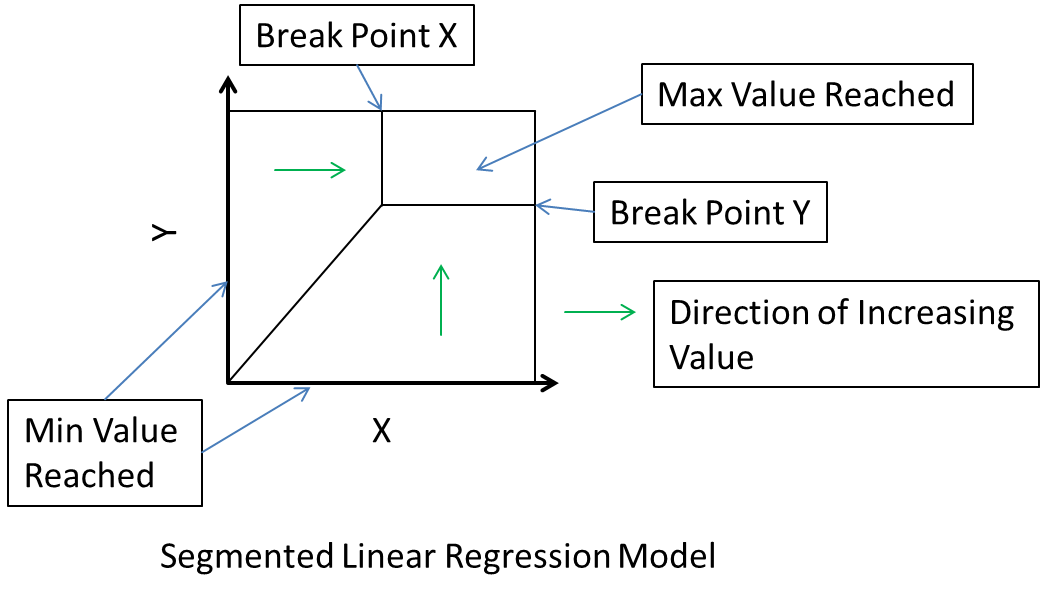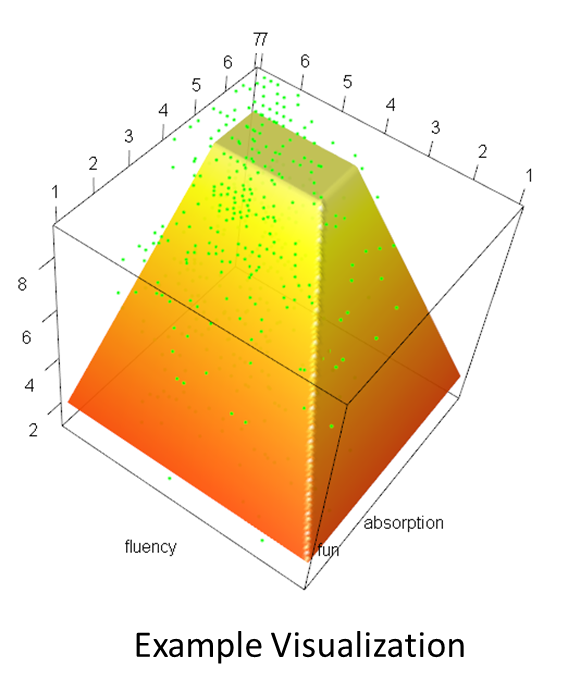# Cowbell: Segmented Linear Regression as Response Surface

## Overview

This package performs a specific form of segmented linear regression analysis over two independent variables. The visualization of that result resembles the quater segment of a cowbell which gives the package name. This package has been specifically constructed for the case where minimal and maximal values of the two independent variables and of the dependent variable are known a prior. This is usually the case if those values are obtained via Likert scale type questionnaires. The definition domain of the resulting regression function is sketched in the following picture.The model of the regression function contains 4 degrees of freedom.

When one of the two independent variables is at their minimal value a predefined minimal value (1st degree of freedom) for the dependent variable is returned. When both independent variables are over their breakpoint value (2nd and 3rd degree of freedom) a defined maximum value (4th degree of freedom) is returned. In the two indicated regions of the definition domain there is a linear increase in value which depends on the smaller of the two independent variables in relation to the breakpoint.

The model takes some time to compute as a gradient optimizer is used to compute the non linear optimization problem.

## Application Conditions

This regression model may be worth trying under the following conditions:

1. There is one dependent variable depending on two independent ones. The minimal and maximal values of those variables are known a prior. This is usually the case if they have been obtained with a Likert scale type questionnaire.
2. In general one expects in increase of the dependent variable if the independent variables are increased.
3. The dependent variable depends mostly on the smaller of the two independent variables.
4. There is a saturation point where increasing an independent variable does not give any more benefit on the dependent one.

## Usage procedure

As a first step a concept is defined. The concept contains the formula of the regression model to be calculated and the minimal and maximal values of the variables included. The formula only contains one dependent and two independent variables. Afterwards follow the minimal and maximal values of the same variables in the same sequence. If one of those values is omitted the corresponding minimum or maximum found in the data set will be used.

concept<-generateCowbellConcept(Fun ~ Fluency * Absorption, 1, 9, 1, 7, 1, 7)

Fun is here measured in the range of 1 … 9 and Fluency and Absorption in the range of 1..7. In a second step that concept then gets applied to a data set. Assuming we apply it to the data set allFun:

test<-generateCowbell(concept, allFun)

As additional parameters the number of iteration steps and the learning rate can be specified. Feel free to experiment with them if they can give you a better result. Increasing the iteration steps results in longer computation times.

The next interesting command for the result is the summary

summary(test)

which results in an output like the following:

## Working on full model.
## Working on model without breakpoint.
## Base concept:
## ===================================================================
## Formula:  Fun ~ Fluency * Absorption
## Fluency  :  1  ...  7
## Absorption  :  1  ...  7
## Fun  :  1  ...  9
##
## Base definition segmented two dimensional linear regression:
## ===================================================================
## Minimal Value dependend variable  Fun :  1.970386
## Maximal Value dependend variable  Fun :  7.638431
## Breaking point of independend variable  Fluency :  4.386884
## Breaking point of independend variable  Absorption :  5.565985
##
## Computation of  Fun :
## ===================================================================
## Fun<-function(Fluency, Absorption)
## {
##   if ((Fluency >= 4.38688353208366) && (Absorption >= 5.56598516657318))
##      return (7.63843073483172)
##   if (Fluency * (-4.56598516657318) + Absorption * 3.38688353208366 > -1.17910163448952)
##      return (0.296857302471897 + Fluency * 1.67352822993063)
##   else
##      return (0.729022497777713 + Absorption * 1.24136303462482)
## }
##
##
## Statistics:
## ===================================================================
## R Squared: 0.3843271
## F-Statistic (comparison constant function): 167.7977  on  3  and  809  DF p-value: 1.436796e-84
##
## ===================================================================
## No breaking point - reduced model
## ===================================================================
## Minimal Value dependend variable  Fun :  2.517464
## Maximal Value dependend variable  Fun :  9
## Computation of  Fun :
## ===================================================================
## Fun<-function(Fluency, Absorption)
## {
##   if (Fluency * (-6) + Absorption * 6 > 0)
##      return (1.43704127615332 + Fluency * 1.08042267483524)
##   else
##      return (1.43704127615332 + Absorption * 1.08042267483524)
## }
##
##
## Statistics:
## ===================================================================
## R Squared: 0.3695049
## F-Statistic (comparison full model against reduced): 9.738247  on  2  and  809  DF p-value: 6.619721e-05

First the output contains the information that was provided in the concept (the formula and minimal / maximal values). Then follows the specification of the values contained in the four degrees of freedom. Afterwards the source code for an explicit R function that computes those values is given. Then the R squared and the F-Statistics of the model compared to a constant function (average) is computed.

In order to estimate the significance of the breakingpoint an additional model with two fewer degrees of freedom is computed. In that model the breaking point is artificially set to the predefined maximal values of the independent variables. The same values as for the full model are also computed here. The difference is in the F-Statistics. In this case the full model is compared to this reduced model with two fewer degrees of freedom to test for the significance of the breaking point.

A visualization of the resulting function can be obtained by

plot(test)

which results in a visualization like the following:## Additional commands

Also the following generic functions that are often used in R for prediction functionality are implemented:

• fitted
• residuals
• predict